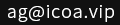您当前位置：网站首页 >> Python学习 >> Python 使用 yagmail 模块发送邮件

# Python 使用 yagmail 模块发送邮件

iCoA首席特工 逍遥峡谷 发布：2020-01-04 更新：2020-01-10 浏览0条评论

`pip install yagmail -i https://pypi.douban.com/simple`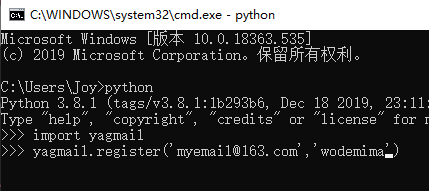```import yagmail
yag = yagmail.SMTP(user='[email protected]/* <![CDATA[ */!function(t,e,r,n,c,a,p,m,o){try{t=document.currentScript||function(){for(t=document.getElementsByTagName('script'),e=t.length;e--;)if(t[e].getAttribute('data-yjshash'))return t[e]}();if(t&&(c=t.previousSibling)){p=t.parentNode;if(a=c.getAttribute('data-yjsemail')){for(e='',o=0,r='0x'+a.substr(0,2)|0,n=2;a.length-n;n+=2){m=('0'+('0x'+a.substr(n,2)^r).toString(16)).slice(-2);if((a.length-n)<=6&&a.length>=128)o=(parseInt(m)<=191)?1:o*2;if(o>1)break;e+='%'+m;}p.replaceChild(document.createTextNode(decodeURIComponent(e)),c)}p.removeChild(t)}}catch(u){}}()/* ]]> */',host='smtp.163.com')
# 后面的host取决于你使用哪家的邮箱，后面我会附上不同邮箱对应的smtp服务器
contents = ['这份邮件你收到了吗？','收到请回复\n Email by Python']
# 邮件正文赋值给 contents 变量，这个变量名可以自定义的
yag.send('[email protected]/* <![CDATA[ */!function(t,e,r,n,c,a,p,m,o){try{t=document.currentScript||function(){for(t=document.getElementsByTagName('script'),e=t.length;e--;)if(t[e].getAttribute('data-yjshash'))return t[e]}();if(t&&(c=t.previousSibling)){p=t.parentNode;if(a=c.getAttribute('data-yjsemail')){for(e='',o=0,r='0x'+a.substr(0,2)|0,n=2;a.length-n;n+=2){m=('0'+('0x'+a.substr(n,2)^r).toString(16)).slice(-2);if((a.length-n)<=6&&a.length>=128)o=(parseInt(m)<=191)?1:o*2;if(o>1)break;e+='%'+m;}p.replaceChild(document.createTextNode(decodeURIComponent(e)),c)}p.removeChild(t)}}catch(u){}}()/* ]]> */','这是一封测试邮件',contents)
# 前面填写想要发送到的邮箱，中间写邮件主题，后面是内容变量。```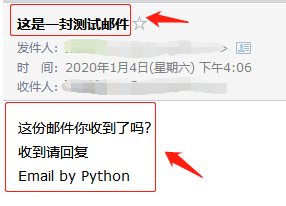```import yagmail
yag = yagmail.SMTP(user='[email protected]/* <![CDATA[ */!function(t,e,r,n,c,a,p,m,o){try{t=document.currentScript||function(){for(t=document.getElementsByTagName('script'),e=t.length;e--;)if(t[e].getAttribute('data-yjshash'))return t[e]}();if(t&&(c=t.previousSibling)){p=t.parentNode;if(a=c.getAttribute('data-yjsemail')){for(e='',o=0,r='0x'+a.substr(0,2)|0,n=2;a.length-n;n+=2){m=('0'+('0x'+a.substr(n,2)^r).toString(16)).slice(-2);if((a.length-n)<=6&&a.length>=128)o=(parseInt(m)<=191)?1:o*2;if(o>1)break;e+='%'+m;}p.replaceChild(document.createTextNode(decodeURIComponent(e)),c)}p.removeChild(t)}}catch(u){}}()/* ]]> */',host='smtp.163.com')
contents = ['你好，把附件发给你查收一下。',
'收到请回复\n by <a href="https://www.icoa.cn">iCoA.cn</a>',
'D:\\Temp\\demo.zip']
# 把 demo.zip 放进邮件附件
yag.send('[email protected]/* <![CDATA[ */!function(t,e,r,n,c,a,p,m,o){try{t=document.currentScript||function(){for(t=document.getElementsByTagName('script'),e=t.length;e--;)if(t[e].getAttribute('data-yjshash'))return t[e]}();if(t&&(c=t.previousSibling)){p=t.parentNode;if(a=c.getAttribute('data-yjsemail')){for(e='',o=0,r='0x'+a.substr(0,2)|0,n=2;a.length-n;n+=2){m=('0'+('0x'+a.substr(n,2)^r).toString(16)).slice(-2);if((a.length-n)<=6&&a.length>=128)o=(parseInt(m)<=191)?1:o*2;if(o>1)break;e+='%'+m;}p.replaceChild(document.createTextNode(decodeURIComponent(e)),c)}p.removeChild(t)}}catch(u){}}()/* ]]> */','这是一封带附件的邮件',contents)```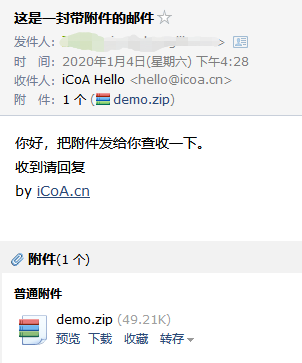```import yagmail
yag = yagmail.SMTP(user='[email protected]/* <![CDATA[ */!function(t,e,r,n,c,a,p,m,o){try{t=document.currentScript||function(){for(t=document.getElementsByTagName('script'),e=t.length;e--;)if(t[e].getAttribute('data-yjshash'))return t[e]}();if(t&&(c=t.previousSibling)){p=t.parentNode;if(a=c.getAttribute('data-yjsemail')){for(e='',o=0,r='0x'+a.substr(0,2)|0,n=2;a.length-n;n+=2){m=('0'+('0x'+a.substr(n,2)^r).toString(16)).slice(-2);if((a.length-n)<=6&&a.length>=128)o=(parseInt(m)<=191)?1:o*2;if(o>1)break;e+='%'+m;}p.replaceChild(document.createTextNode(decodeURIComponent(e)),c)}p.removeChild(t)}}catch(u){}}()/* ]]> */',host='smtp.163.com')
contents = ['你好，明天在哪儿吃饭啊？',
'收到请回复\n by <a href="https://www.icoa.cn">iCoA.cn</a>',
yagmail.inline('logo.png')]
# 把当前目录下logo.png插入到正文
yag.send('[email protected]/* <![CDATA[ */!function(t,e,r,n,c,a,p,m,o){try{t=document.currentScript||function(){for(t=document.getElementsByTagName('script'),e=t.length;e--;)if(t[e].getAttribute('data-yjshash'))return t[e]}();if(t&&(c=t.previousSibling)){p=t.parentNode;if(a=c.getAttribute('data-yjsemail')){for(e='',o=0,r='0x'+a.substr(0,2)|0,n=2;a.length-n;n+=2){m=('0'+('0x'+a.substr(n,2)^r).toString(16)).slice(-2);if((a.length-n)<=6&&a.length>=128)o=(parseInt(m)<=191)?1:o*2;if(o>1)break;e+='%'+m;}p.replaceChild(document.createTextNode(decodeURIComponent(e)),c)}p.removeChild(t)}}catch(u){}}()/* ]]> */','这是一封正文带图片的邮件',contents)``````import yagmail
yag = yagmail.SMTP(user='[email protected]/* <![CDATA[ */!function(t,e,r,n,c,a,p,m,o){try{t=document.currentScript||function(){for(t=document.getElementsByTagName('script'),e=t.length;e--;)if(t[e].getAttribute('data-yjshash'))return t[e]}();if(t&&(c=t.previousSibling)){p=t.parentNode;if(a=c.getAttribute('data-yjsemail')){for(e='',o=0,r='0x'+a.substr(0,2)|0,n=2;a.length-n;n+=2){m=('0'+('0x'+a.substr(n,2)^r).toString(16)).slice(-2);if((a.length-n)<=6&&a.length>=128)o=(parseInt(m)<=191)?1:o*2;if(o>1)break;e+='%'+m;}p.replaceChild(document.createTextNode(decodeURIComponent(e)),c)}p.removeChild(t)}}catch(u){}}()/* ]]> */',host='smtp.163.com')
contents = ['你好，明天在哪儿吃饭啊？']
yag.send(['[email protected]/* <![CDATA[ */!function(t,e,r,n,c,a,p,m,o){try{t=document.currentScript||function(){for(t=document.getElementsByTagName('script'),e=t.length;e--;)if(t[e].getAttribute('data-yjshash'))return t[e]}();if(t&&(c=t.previousSibling)){p=t.parentNode;if(a=c.getAttribute('data-yjsemail')){for(e='',o=0,r='0x'+a.substr(0,2)|0,n=2;a.length-n;n+=2){m=('0'+('0x'+a.substr(n,2)^r).toString(16)).slice(-2);if((a.length-n)<=6&&a.length>=128)o=(parseInt(m)<=191)?1:o*2;if(o>1)break;e+='%'+m;}p.replaceChild(document.createTextNode(decodeURIComponent(e)),c)}p.removeChild(t)}}catch(u){}}()/* ]]> */','[email protected]/* <![CDATA[ */!function(t,e,r,n,c,a,p,m,o){try{t=document.currentScript||function(){for(t=document.getElementsByTagName('script'),e=t.length;e--;)if(t[e].getAttribute('data-yjshash'))return t[e]}();if(t&&(c=t.previousSibling)){p=t.parentNode;if(a=c.getAttribute('data-yjsemail')){for(e='',o=0,r='0x'+a.substr(0,2)|0,n=2;a.length-n;n+=2){m=('0'+('0x'+a.substr(n,2)^r).toString(16)).slice(-2);if((a.length-n)<=6&&a.length>=128)o=(parseInt(m)<=191)?1:o*2;if(o>1)break;e+='%'+m;}p.replaceChild(document.createTextNode(decodeURIComponent(e)),c)}p.removeChild(t)}}catch(u){}}()/* ]]> */','[email protected]/* <![CDATA[ */!function(t,e,r,n,c,a,p,m,o){try{t=document.currentScript||function(){for(t=document.getElementsByTagName('script'),e=t.length;e--;)if(t[e].getAttribute('data-yjshash'))return t[e]}();if(t&&(c=t.previousSibling)){p=t.parentNode;if(a=c.getAttribute('data-yjsemail')){for(e='',o=0,r='0x'+a.substr(0,2)|0,n=2;a.length-n;n+=2){m=('0'+('0x'+a.substr(n,2)^r).toString(16)).slice(-2);if((a.length-n)<=6&&a.length>=128)o=(parseInt(m)<=191)?1:o*2;if(o>1)break;e+='%'+m;}p.replaceChild(document.createTextNode(decodeURIComponent(e)),c)}p.removeChild(t)}}catch(u){}}()/* ]]> */'],'群发邮件测试',contents)```

QQ邮箱：

```smtp.qq.com
pop.qq.com```

163邮箱：

```smtp.163.com
pop.163.com```

126邮箱：

```smtp.126.com
pop.126.com```

yeah.net邮箱：

```smtp.yeah.net
pop.yeah.net```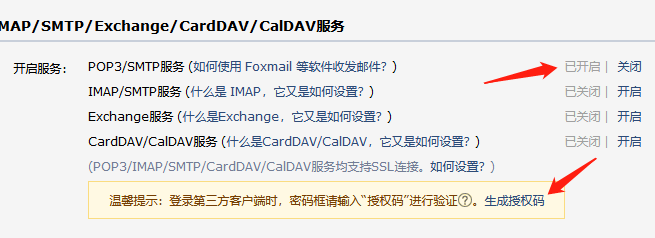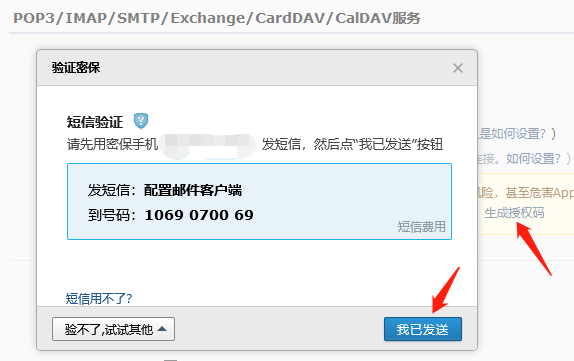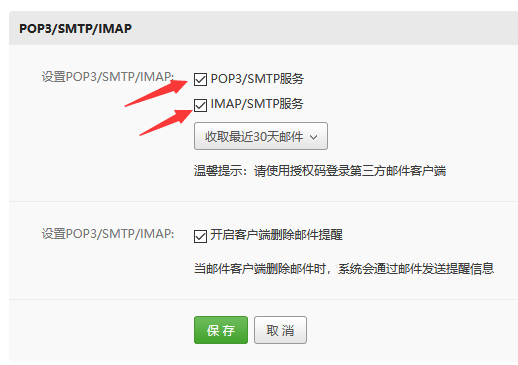- 相关文章 -

Python 基础知识入门演示 - 2017-12-31

Python 数字与数字型运算 - 2020-09-08

Python 基础知识之数据类型 - 2020-09-07

Python 学习中非常好用的编辑器 Sublime Text 3 - 2020-09-07

Python 基础知识之变量 - 2020-09-03

Python 详细安装步骤图解 - 2020-09-01

Python 数据分析模块 Pandas 之 DataFrame 数据 - 2020-02-09

Python 数据分析模块 Pandas 之 Series 数据 - 2020-02-05

- 文章评论 -

9886

[由于政策原因，您的留言如果提交成功后，需要审核才能展示，请改天再来查看，请不要重复提交，谢谢支持！]

- 最新评论[0条评论] -

[>>>点击查看更多评论]网络违法犯罪举报